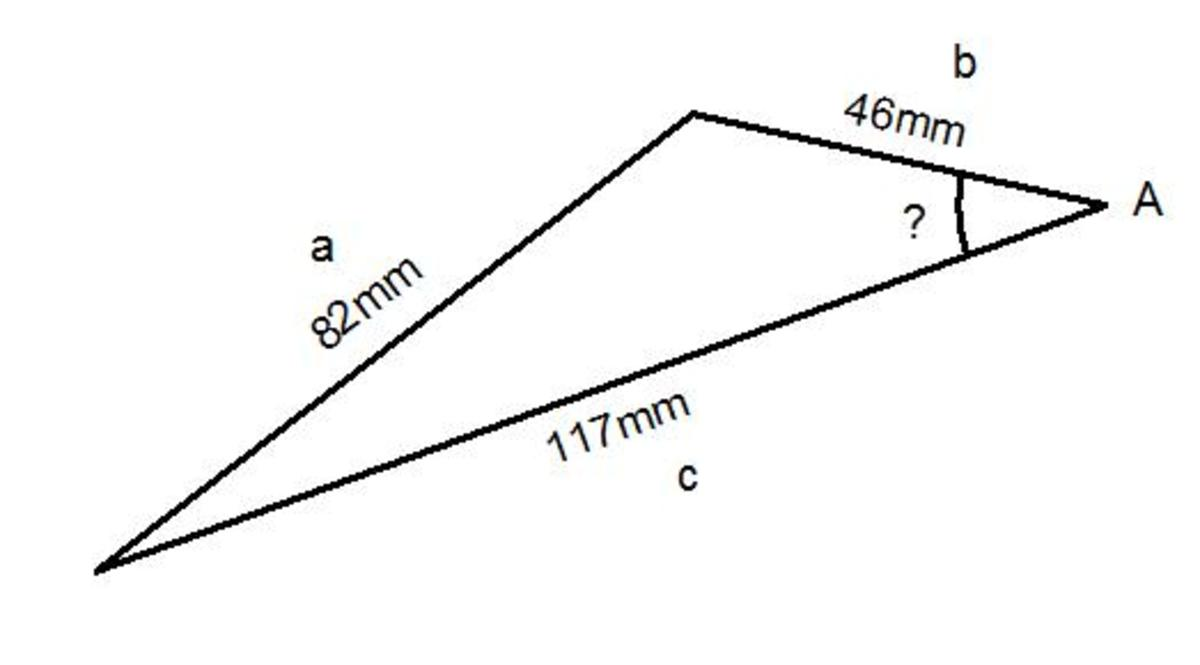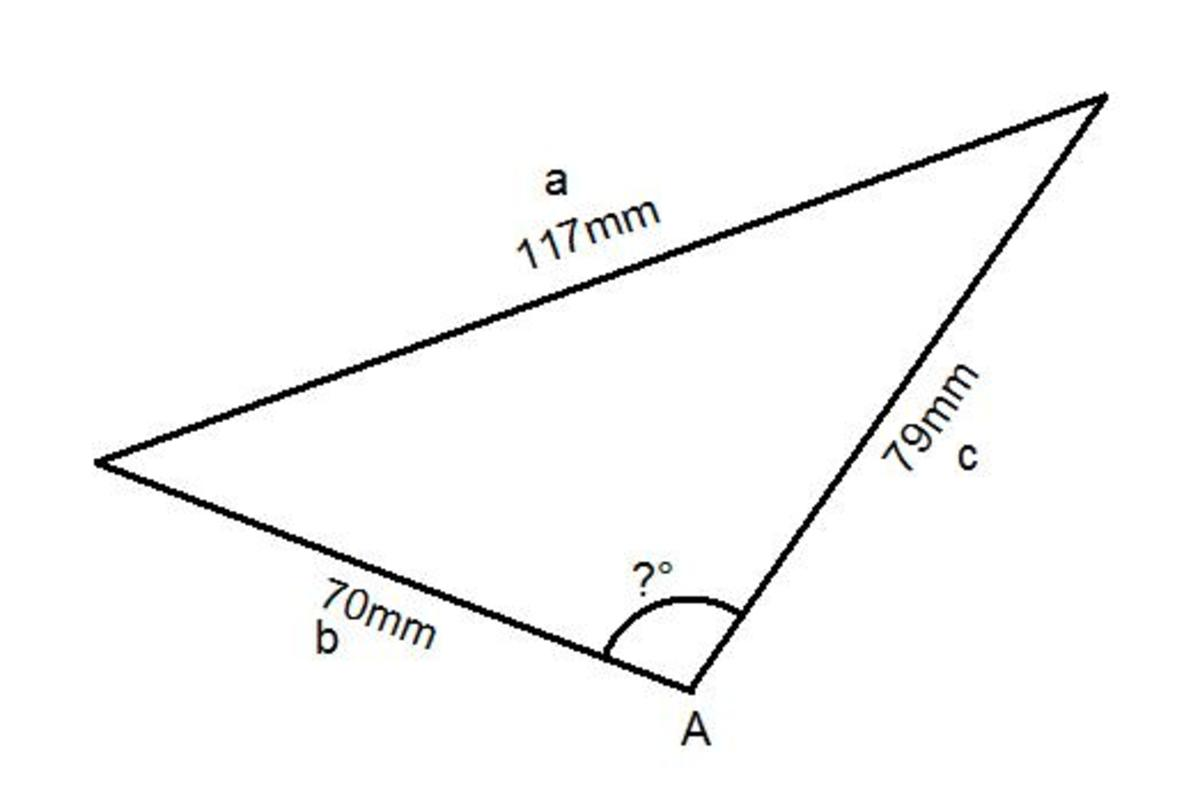Updated date:

# The Cosine Rule. How to Work out an Angle Inside any Triangle Given 3 Side Lengths.

Author:

When you are given all of the 3 sides of a triangle then any angle inside the triangle can be found by applying the cosine rule (the angle version is easiest to apply). The formula for this is:

CosA = (b² + c² - a²)/(2bc)

A is the angle that you are finding and a is the side that is opposite the angle.

b and c are the lengths of the other 2 sides of the triangle. It doesn’t matter which way around you put b and c as you will get the same answer either way.

You can use a² = b² + c² -2bcCosA but this is harder to apply to questions that involve working out an angle.

Let’s take a look at a couple of questions that involve working out an angle using the cosine rule.

Example 1

Work out the missing angle in this triangle.Since you given all 3 sides of the triangle and you need to work out an angle then the problem can be solved using the cosine rule.

First of all label up your triangle:

Since you are working out the angle call this big A.

Therefore, the side opposite this angle you are finding is little a.

The two other sides can be called b and c.

All you need to do know is apply the Cosine rule:

Substitute A = ?, a = 82, b = 46 and c = 117 into the formula above:

CosA = (b² + c² - a²)/(2bc)

Cos? = (82² + 46² - 117²)/(2×46×117)

Cos? = 9081/10764 (all you need to do next is cos inverse this fraction to convert it to an angle)

? = 32.5

Example 2

Work out the missing angle in this triangle.Since you given all 3 sides of the triangle and you need to work out an angle then the problem can be solved using the cosine rule.

First of all label up your triangle:

Since you are working out the angle call this big A.

Therefore, the side opposite this angle you are finding is little a.

The two other sides can be called b and c.

All you need to do know is apply the Cosine rule:

Substitute A = ?, a = 117, b = 70 and c = 79 into the formula above:

CosA = (b² + c² - a²)/(2bc)

Cos? = (70² + 79² - 117²)/(2×70×79)

Cos? = -2548/11060 (all you need to do next is cos inverse this fraction to convert it to an angle)

? = 103.3⁰ (rounded to 1 decimal)

As you can see using this version of the cosine rule is actually quite easy to work out an angle. Just take care labelling up your triangle and subbing your values into the cosine rule formula. Also make sure that you have cos inversed your final answer.